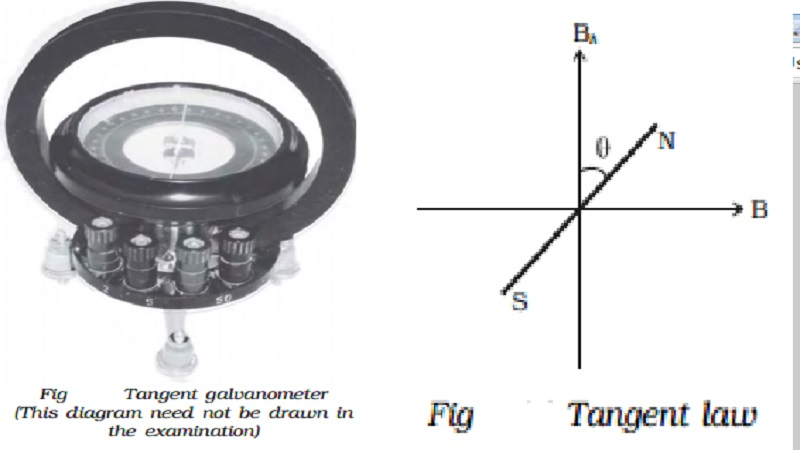Home | | Physics | | Physics | Tangent galvanometer : Theory and Construction of Tangent galvanometer

# Tangent galvanometer : Theory and Construction of Tangent galvanometerTangent galvanometer : Tangent galvanometer is a device used for measuring current. It works on the principle of tangent law. A magnetic needle suspended at a point where there are two crossed fields at right angles to each other will come to rest in the direction of the resultant of the two fields.

Tangent galvanometer

Tangent galvanometer is a device used for measuring current. It works on the principle of tangent law. A magnetic needle suspended at a point where there are two crossed fields at right angles to each other will come to rest in the direction of the resultant of the two fields.## Construction

It consists of a circular coil of wire wound over a non magnetic frame of brass or wood. The vertical frame is mounted on a horizontal circular turn table provided with three levelling screws. The vertical frame can be rotated about its vertical diameter.

There is a small upright projection at the centre of the turn table on which a compass box is supported.

The compass box consists of a small pivoted magnet to which a thin long aluminium pointer is fixed at right angles. The aluminium pointer can move over a circular scale graduated in degrees. The scale consists of four quadrants. The compass box is supported such that the centre of the pivoted magnetic needle coincides with the centre of the coil. Since the magnetic field at the centre of the coil is uniform over a very small area, a small magnetic needle is used so that it remains in an uniform field even in deflected position. Usually the coil consists of three sections of 2,5 and 50 turns, which are of different thickness, used for measuring currents of different strength.

## Theory

When the plane of the coil is placed parallel to the horizontal component of Earth’s magnetic induction (Bh) and a current is passed through the coil, there will be two magnetic fields acting perpendicular to each other : (1) the magnetic induction (B) due to the current in the coil acting normal to the plane of the coil and (2) the horizontal component of Earth’s magnetic induction (Bh) (Fig 3.14).Due to these two crossed fields, the pivoted magnetic needle is deflected through an angle θ. According to tangent Law,Since the tangent galvanometer is most sensitive at a deflection of 450, the deflection has to be adjusted to be between 300 and 600.

Study Material, Lecturing Notes, Assignment, Reference, Wiki description explanation, brief detail

Related Topics